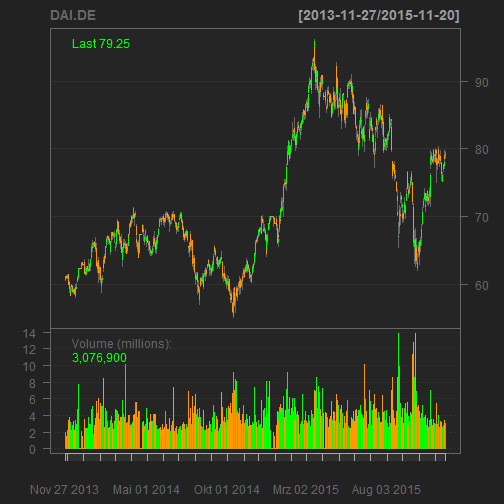# Revisiting barrier bond

In Analyse einer Barriere-Anleihe I was estimating the present value of a barrier bond emitted by Berliner Landesbank, which referred to the stock price of Daimler: The payout would be 100, if the price was either allways above the barrier of 70%, or, if it dipped below the barrier, but closed above the initial price at the maturity of the bond. In every other case, the buyer would get the value of the stock at maturity. For details on the bond, go here.

Using stochastic modelling on a 13 year history of the Daimler stock price, I estimated a present value at offering of 98.6% assuming a risk free rate of 2% for the 2 year maturity (I checked the sensitivity of the result to the risk free rate, but even at a -0.5% the mean present value is at 99.4%, so a slight loss).

Today I wanted to check what the actual payout would have been.

payoutEnd = function(basis, barriere, kurse)
{
basis = as.numeric(basis)
barriere = as.numeric(barriere)
kurse = as.numeric(kurse)
if(all(kurse >= barriere))
{
res = kurse
} else if( last(kurse)>basis)
{
res = kurse
} else
{
res = last(kurse)
}
return(res)
}

##  "DAI.DE"Okay, so it seems the price never went below the barrier of 42.462, and the final payout was 100%:

payoutEnd(1,0.7, DAI.DE[,3]/DAI.DE[1,3])

##  1


So, using hindsight, this would have been a good investment given the 7% coupon.

# So I finally understood Monty Hall

Lately I have been binge-watching Mythbusters, and one of the more curios myths they took on was the Monty Hall problem. The Monty Hall problem is named after a US TV show, were the candidate had the chance to win whatever price was behind one of three doors, where the other two doors had no price. The twist is that after the candidate choose, the moderator would show what was behind one of the other two doors, obviously one, where no price is, and the candidate now had the chance to switch the door.

Now, intuitively one would say that being shown what is behind a door will not change the chances, and the candidate has a 1 in 3 chance to win the price. Now the myth is, that switching the door will increase the chance to win substantially.

One might say, this is not really a myth, as it can be shown statistically to be true. But I am bad at combinatoric, so after seeing in Mythbusters how far ahead the switching strategy is, I wanted to redo their experiment as a Monte Carlo simulation.

First, we set up the experiment, and sample the winning doors, and the initial selection by the candidate.

# monty hall problem

n = 100000

prices = sample(3,n,1)

selected = sample(3,n,1)

df = data.frame(prices = prices,
selected = selected,
shown = NA,
wins_stay = NA,
wins_switch = NA)


##   prices selected shown wins_stay wins_switch
## 1      2        1    NA        NA          NA
## 2      3        2    NA        NA          NA
## 3      3        2    NA        NA          NA
## 4      3        2    NA        NA          NA
## 5      1        1    NA        NA          NA
## 6      2        3    NA        NA          NA


Next, we define how the moderator has to choose, which door to show in each case. And this is the first hint to why the likelihood to win is higher if the candidate switches: We need to differ between the cases were the candidate chose the winning door or not, because in the case of the candidate choosing a losing door, the door to be opened by the moderator is predetermined – it’s the one which is not winning.

shown = apply(df, 1, function(x){
x = unlist(x)

# x - winning door, x - choosen door
# candidate choose winning door

if(x==x){
return(sample((1:3)[-x],1))
} else {
return((1:3)[-c(x, x)])
}
})
df$shown = shown head(df)  ## prices selected shown wins_stay wins_switch ## 1 2 1 3 NA NA ## 2 3 2 1 NA NA ## 3 3 2 1 NA NA ## 4 3 2 1 NA NA ## 5 1 1 2 NA NA ## 6 2 3 1 NA NA  Next, we calculate the winning likelihood, if the candidate always stays with the initial selection selected_stay = selected df$wins_stay = prices == selected_stay

##   prices selected shown wins_stay wins_switch
## 1      2        1     3     FALSE          NA
## 2      3        2     1     FALSE          NA
## 3      3        2     1     FALSE          NA
## 4      3        2     1     FALSE          NA
## 5      1        1     2      TRUE          NA
## 6      2        3     1     FALSE          NA

sum(df$wins_stay)/n  ##  0.33196  It’s not very surprising that the percentage is 1 in 3, which is the initial likelihood without any additional information. Finally, we have to compute the door the candidate chooses if he switches. selected_switch = apply(df,1,function(x){ x = unlist(x) (1:3)[!(1:3)%in%c(x, x)] }) df$wins_switch = prices == selected_switch

##   prices selected shown wins_stay wins_switch
## 1      2        1     3     FALSE        TRUE
## 2      3        2     1     FALSE        TRUE
## 3      3        2     1     FALSE        TRUE
## 4      3        2     1     FALSE        TRUE
## 5      1        1     2      TRUE       FALSE
## 6      2        3     1     FALSE        TRUE

sum(df\$wins_switch)/n

##  0.66804


Following the switching strategy, the candidates chances are 2 in 3, which counter-intuitively is quite logical: The candidate will loose in each case where his initial selection was correct (1 in 3), but will win in each case where his initial selection was wrong (2 in 3).

Oh, and here is a nice clip explaining it much better: